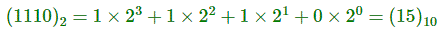# how to convert binary digit into decimal and decimal to binary??

54 views## Conversion from Binary to Decimal

Expand the number given in binary form in the power of 2 and sum the values, the result which we will get will be in the decimal form. For example-## Conversion from Decimal to Binary

Divide the number by 2 and take only the remainder, if division is completed than take only the remainder which gives the binary number.

Example∴ (87)10 = (1110101)2